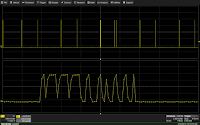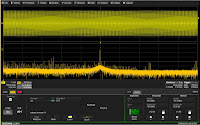## You need to test, we're here to help.

You need to test, we're here to help.

## 01 October 2015

### Taking Best Advantage of Oscilloscopes' Long MemoryFigure 1: Maintaining the maximum sample rate over more timebase settings is possible with long memory
Two very important considerations when choosing a digital oscilloscope are the length of the acquisition memory and the amount of RAM available for processing of the raw data. Note that acquisition memory and RAM are not the same things, but they are still both important. The amount of acquisition memory often determines the fidelity with which an oscilloscope can record a signal. But that's only the first step; it's the instrument's processing horsepower is the key to finding signal abnormalities and characterizing circuit performance.

The maximum time window that a digital oscilloscope can capture at a given sampling rate is:

Time window = memory length / sampling rate

where acquisition memory length is the maximum number of samples that can be captured in the data acquisition memory. Because the acquisition memory length is of a finite amount, the only way to capture longer time windows is to lower the sampling rate  (Figure 1). For example, an oscilloscope such as the Teledyne LeCroy WaveSurfer 3000 with 10 Mpoints of memory and a sampling rate of 500 Msamples/s (2-ns sampling period) can capture a total time window of 0.02 seconds at that sampling rate.Figure 2: An example of an undersampled CANbus signal
If you wanted to see a 0.04-second signal using 10 Mpoints, the points would have to be stretched further apart to 25 Msamples/s (20-ns sampling period). This means that the timing resolution is degraded by a factor of 20; many signal details would be lost. Plus, any frequency >12.5 MHz (half the sampling rate) would be aliased (Figure 2).

Some digital oscilloscope users would believe that the analog-to-digital converter (ADC) in their instruments determine its sampling rate. The ADC determines the maximum sampling rate, but don't discount the role played by the length of the acquisition memory. A long acquisition memory allows you to maintain high sampling rates at longer capture times. As much as 1.5 Gpoints of memory is available in Teledyne LeCroy's state-of-the-art LabMaster 10 Zi-A oscilloscope. This allows for a capture time of 6.25 ms with a sampling rate of 240 Gsamples/s!

Let's say your oscilloscope contains a modest 2 million sample points of memory per channel. In the example given above, a full 4 ms can be captured at 2 ns/sample using 2 million points. At the end of the day, an oscilloscope that gives 2 million points to 4 ms worth of signal capture will give you 40 times better timing resolution, a much better view of the signal, and more usable bandwidth than one that spreads the same signal over 100,000 points.

#### Using Long Memory to Spot Signal AnomaliesFigure 3: A 1-GHz clock modulated by a 31-kHz SSC
The most common use for long memory is to fill the need to simultaneously capture high-speed and low-speed signals. For example, Figure 3 shows a 1-GHz clock being modulated by a 31-kHz spread-spectrum clock (SSC). You need enough sampling rate to capture the 1-GHz clock and also have a long enough capture time to see at least one full period of the SSC.Figure 4: At lower right, zoom trace Z3 shows a single output pulse from a motor drive's inverter
Another good example of the utility of long memory is in motor-drive applications. The gates of the motor drive's inverter are driven by a PWM signal and thus have a pulsed output. The duration of these pulses is very short and require a high sampling rate to capture. However, the actual spinning of the motor and its dynamic behaviors (startup, overload, dynamic load change, and so on) happen very slowly, in a period that is perhaps on the order of seconds. Again, you need the high sampling rate to capture the short pulses and the long memory to see the motor's dynamic behavior. Figure 4 shows the output of a motor drive as the motor starts up. There is a 200,000:1 zoom ratio from the total capture to the Z3 trace at lower right, which displays an individual inverter output pulse.

#### Long Memory and the Frequency Domain

Many modern digital oscilloscopes provide fast-Fourier transform (FFT) capabilities. Because the FFT in an oscilloscope comes from a set of discrete points (with sampling period Δt), the information in the frequency domain is also a discrete set of points (with spacing of Δf).

Resolution in the frequency domain is determined by two factors: the frequency span being measured and the number of points within that span. Nyquist's theorem determines the measurable range of frequencies: from DC to one-half of the sampling rate at which the data was captured. A Fourier transform of an array of N time-domain data points produces N/2 frequency-domain points within the range of frequencies from DC to the Nyquist frequency. Thus, the frequency resolution (Δf) of the FFT is:

(1/2) sampling rate
(1/2) number of points input to the FFT algorithmFigure 5: This screen capture of an FFT uses only 12.5 kS, resulting in broad frequency peaks
The two factors of 1/2 cancel, giving a resolution equal to the sampling rate divided by the number of points input to the FFT. Obviously, it is important to capture the data at a high sampling rate. A long acquisition memory plays a key role, because it enables the instrument to have a high sampling rate for a longer period of time, which in turn facilitates inputting more data points to the FFT algorithm.

However, simply capturing the points isn't the whole answer. The oscilloscope must have enough processing horsepower to compute the FFT on a long data array. Some oscilloscopes that have appeared on the market limit the FFT processing to some number of points that may be far fewer than the number the instrument is able to capture. The result is an FFT with greatly reduced frequency resolution.Figure 6: The same FFT shown in Figure 5 now comprises 1.25 MS, clearly revealing two frequency peaks
To illustrate the point, Figure 5 is a screen capture of an FFT taken on a waveform using 12.5 kS. In this image, we see very broad frequency peaks due to the low sampling resolution. If we perform a second FFT of the same waveform, this time using 1.25 MS instead of 12.5 kS (Figure 6), the difference in frequency resolution is a factor of 100. Now we clearly see that there are two frequency peaks at closely spaced frequencies. Those two peaks could not be resolved in the first FFT of Figure 5.

Stay tuned for more on how you can best make use of your digital oscilloscope's deep acquisition memory in future posts.